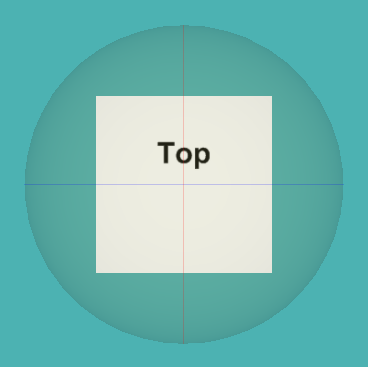This chapter illustrates design patterns and best practices for authoring different geometries through a collection of interesting 3D shapes.

See: Description

• Class Summary
Class Description
AxisLinesRGB
AxisLinesRGB shows 3 red-green-blue (RBB) perpendicular lines suitable as X-Y-Z axes.
CapsuleComparison
CapsuleComparison shows three capsules, using polygons/lines/points rendering, each a hollow cylinder with hemispherical end caps.
CapsuleGenerator
Capsule has top and bottom hemispheres with connected sides, with adjustable parameters in the contained Script fields.
Catenary
A catenary is the curve that an idealized hanging chain or cable assumes under its own weight when supported only at its ends.
CubeWithLabeledSides
Cube-shaped test model with faces on each side individually labeled.
CylinderIndexedFaceSet
Construct a Cylinder using IndexedFaceSet.
Dodecahedron
Dodecahedron is a 12-sided polyhedron with 30 edges, 20 vertices and 12 pentagonal faces.
ExtrusionEdgeCases
Scene incorporating extrusion edge cases.
ExtrusionExamplesTest
Extrusion examples for testing and debugging.
Hemisphere
Hemisphere is a half sphere.
Icosahedron
Icosahedron is a polyhedron with 12 vertices and, 20 faces, where a regular icosahedron is a Platonic solid.
IcosahedronSubdivisionLevel1
Icosahedron is a polyhedron with twenty faces, subdivided to level 1, where all 42 vertices and 80 faces produce regular (equilateral) triangles.
IcosahedronSubdivisionLevel2
Icosahedron is a polyhedron with twenty faces, subdivided to level 2, where all 162 vertices and 320 faces produce regular (equilateral) triangles.
IcosahedronSubdivisionLevel3
Icosahedron is a polyhedron with twenty faces, subdivided to level 3, where all 642 vertices and 1280 faces produce regular (equilateral) triangles.
IcosahedronSubdivisionLevel4
Icosahedron is a polyhedron with twenty faces, subdivided to level 4, where all 2562 vertices and 3366 faces produce regular (equilateral) triangles.
IcosahedronSubdivisionLevel5
Icosahedron is a polyhedron with twenty faces, subdivided to level 5, where all 10242 vertices and 20480 faces produce regular (equilateral) triangles.
MobiusExtrusion
Mobius (or Mobius) is a surface with only one side and only one boundary component.
Octahedron
Octahedron is an 8-sided polyhedron with 6 vertices, 8 triangular faces and 12 edges.
ParallelepipedExamples
Example use of external prototype declaration for Parallelepiped, which is a 3D figure formed by six parallelograms.
ParallelepipedPrototype
Reusable prototype for creating a Parallelepiped, which is a 3D figure formed by six parallelograms; also sometimes referred to as a rhomboid.
RoundedRectangle2D
RoundedRectangle2D shows and IndexedFaceSet shape for a flat RoundedRectangle.
RoundedRectangleComparison
RoundedRectangleComparison shows three RoundedRectangle shapes using polygons/lines/points rendering.
RoundedRectanglePrimitives
Illustrates how to build rounded rectangle using only primitive nodes.
SphereWithThreeCircumferenceRings
Semitransparent Sphere with circumference circles on x-y-z planes to facilitate user rotation of an object, suitable for use as rotation widget.
StairStepPrototype
Build one or more stair steps, in a straight line.
Teapot
A watertight (solid) Utah Teapot Model converted from obj format.
Teapots
Four watertight (solid) Utah Teapot Models converted from obj format.
Tetrahedron
Tetrahedron is an 8-sided pyramidal polyhedron with 4 vertices, 4 triangular faces and 6 edges.
TriangleBlackLines
Simple Triangle with black lines, illustrating default line rendering.
TriangleWithNumbers
Simple Triangle with labeled points, where labels always face the user and stay in place with the triangle.

## Package X3dForAdvancedModeling.GeometricShapes Description

This chapter illustrates design patterns and best practices for authoring different geometries through a collection of interesting 3D shapes.The open-source Meshlab and X3D-Edit authoring tools helped create many of these examples. Tools of interest for generating mathematical shapes include:
 Wikipedia: Platonic solid and Geodesic grid Wolfram Mathworld: Geometry X3D-Edit